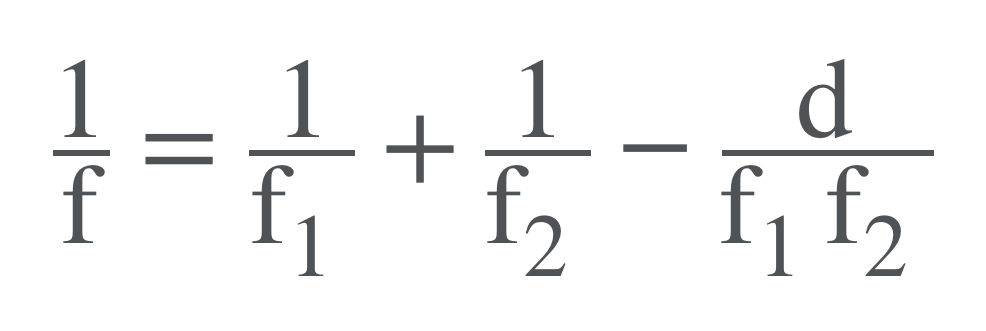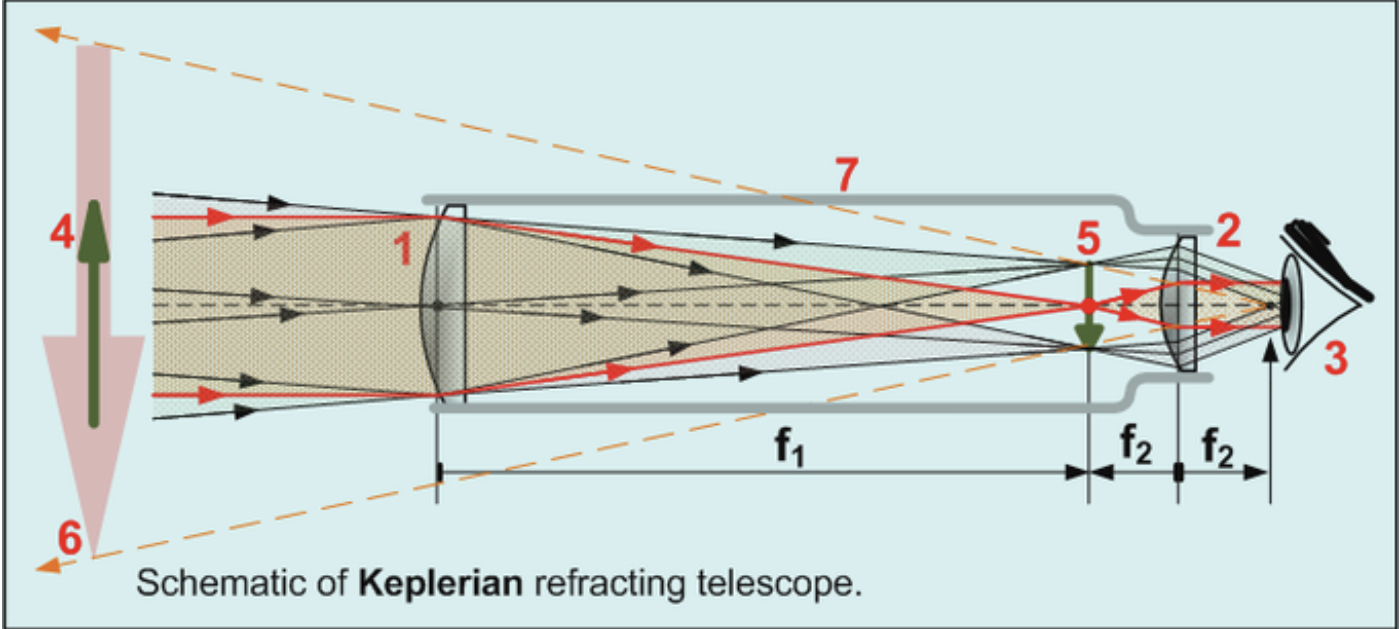Complimentary 1-hour tutoring consultation

MCAT Content / Geometrical Optics / Combination Of Lenses

### Combination of lenses

Topic: Geometrical Optics

An array of simple lenses with a common axis can be used to multiply the magnification of an image. The real image formed by one lens can be used as the object for another lens, combining magnifications.

Lenses in contact: The simplest case is where lenses are placed in contact: if the lenses of focal lengths f1 and f2 are “thin”, the combined focal length f of the lenses is given bySince 1/f is the power of a lens, it can be seen that the powers of thin lenses in contact are additive.

Separated lenses: If two thin lenses are separated in air by some distance d (where d is smaller than the focal length of the first lens), the focal length for the combined system is given byThe distance from the second lens to the focal point of the combined lenses is called the back focal length (BFL).As d tends to zero, the value of the BFL tends to the value of f given for thin lenses in contact.

If the separation distance is equal to the sum of the focal lengths (d = f1+f2), the combined focal length and BFL are infinite. This type of system is called an afocal system since it produces no net convergence or divergence of the beam: transform a parallel beam into another parallel beam (collimated). Although the system does not alter the divergence of a collimated beam, it does alter the width of the beam. The magnification can be found by dividing the focal length of the two lenses.

Two lenses at this separation form the simplest type of optical telescope. A schematic of a simple telescope is a good example of the use of two lenses to focus the image of one lens:Practice Questions

Converging and diverging lenses in a lab

A mirror in an operating room

MCAT Official Prep (AAMC)

Key Points

• If the lenses of focal lengths f1 and f2are “thin”, the combined focal length f of the lenses is given by 1/f=1/f1+1/f2

• If the lenses are separated by some distance d, then the combined focal length is given by 1/f=1/f1+1/f2−d/(f1f2)

• If the separation distance is equal to the sum of the focal lengths (d = f1+f2), the combined focal length is infinite. This type of system is called an afocal system (a simple optical telescope).

Key Terms

afocal system: an optical system that produces no net convergence or divergence of the beam.

Billing Information